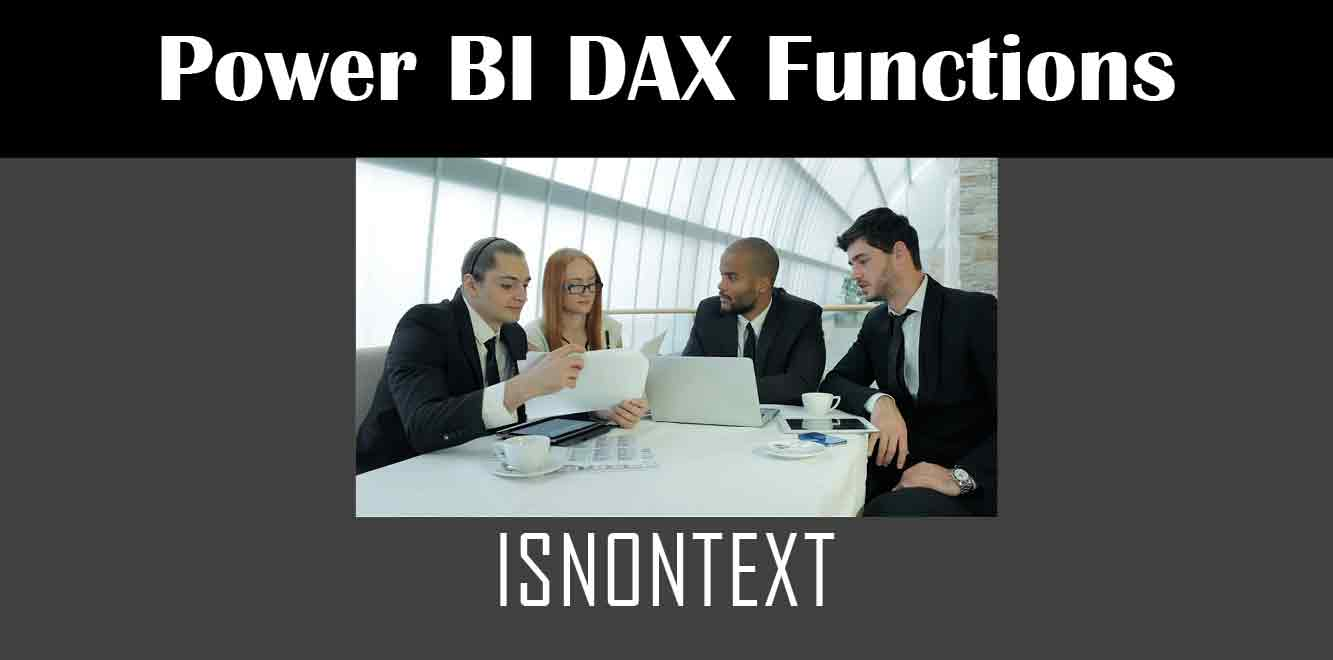# ISNONTEXT

## Y## Understanding the ISNONTEXT Function

The ISNONTEXT function in DAX is used to determine whether a value is not a text string. It returns the value TRUE if the expression is not a text string, and FALSE if it is a text string.

The syntax for the function is as follows:

ISNONTEXT(❰value❱)

``` Here, the value argument can be a column reference, a scalar value, or an expression that returns a value. Examples of Using the ISNONTEXT Function To illustrate the use of the ISNONTEXT function, let's consider some examples. Example 1: Using ISNONTEXT with a Column Reference Suppose we have a table called "Sales" with the following columns: "Product", "Quantity", and "Price". The "Quantity" column contains numerical values while the "Product" column contains text values. We can use the ISNONTEXT function to create a new column that indicates whether the "Product" value is not a text string. To do this, we can use the following formula: ```

Non-Text Product = ISNONTEXT(Sales[Product])

``` This formula will return TRUE for all rows where the "Product" value is not a text string. Example 2: Using ISNONTEXT with a Scalar Value Suppose we have a measure called "Total Sales" that calculates the total sales for a given period. We want to create a new measure called "Total Sales (Text Only)" that calculates the total sales for periods where the "Period" value is a text string. To do this, we can use the following formula: ```

Total Sales (Text Only) = CALCULATE([Total Sales], ISNONTEXT(‘Sales'[Period]))

``` This formula will calculate the total sales only for periods where the "Period" value is a text string. In conclusion, the ISNONTEXT function in DAX is a useful tool for data analysis in Power BI. It allows users to determine whether a value is not a text string, which can be helpful in creating custom calculations and formulas. By using the examples provided above, you can start using the ISNONTEXT function in your own Power BI projects. Power BI DAX Training Courses by G Com Solutions (0800 998 9248)Power BI DAX Intensive Training Course £1,050.00 – £26,550.00 Select optionsContinue Loading DonePower BI DAX Introduction £395.00 – £9,750.00 Select optionsContinue Loading DonePower BI DAX Intermediate £395.00 – £9,750.00 Select optionsContinue Loading DonePower BI DAX Advanced £395.00 – £9,750.00 Select optionsContinue Loading Done Upcoming Courses Contact Us Subject Your Name (required) Company/Organisation Email (required) Telephone Training Course(s) Power BI Intensive TrainingPower BI introduction Power BI IntermediatePower BI AdvancedDAXPower Query MPower BI CertificationPower BI AdministrationPower PlatformPower AutomatePower AppsOTHER Your Message Upload Example Document(s) (Zip multiple files) ```
``` ```
``` ```
``` ```
``` ```
``` ```